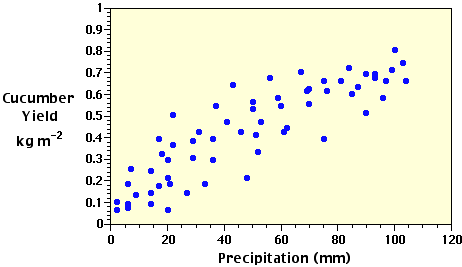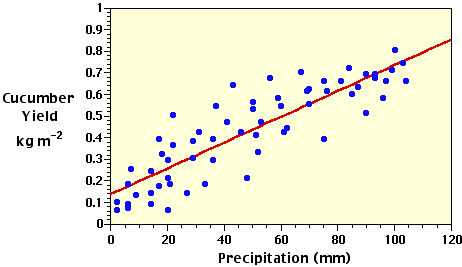## Thursday, December 29, 2011

### Inferential Statistics: Regression and Correlation Part B

(h) Inferential Statistics: Regression and Correlation Part B

S X = 3,050
•  = 49.1935

S Y = 26.62

•  = 0.4294

n = 62

Often the first step in regression analysis is to plot the X and Y data on a graph (Figure 3h-1). This is done to graphically visualize the relationship between the two variables. If there is a simple relationship, the plotted points will have a tendancy to form a recognizable pattern (a straight line or curve). If the relationship is strong, the pattern will be very obvious. If the relationship is weak, the points will be more spread out and the pattern less distinct. If the points appear to fall pretty much at random, there may be no relationship between the two variablesFigure 3h-1: Scattergram plot of the precipitation and cucumber yield data found in Table 3h-1. The distribution of the data points indicates a possible positive linear relationship between the two variables.
The type of pattern (straight line, parabolic curve, exponential curve, etc.) will determine the type of regression model to be applied to the data. In this particular case, we will examine data that produces a simple straight-line relationship (see Figure 3h-1). After selecting the model to be used, the next step is to calculate the corrected sums of squares and products used in a bivariate linear regression analysis. In the following equations, capital letters indicate uncorrected values of the variables and lower-case letters are used for the corrected parameters in the analysis.

The corrected sum of squares for Y:

S y2 = S Y2 -= (0.362 + 0.092 + ... + 0.422) - (26.622) / 62

= 2.7826

The corrected sum of squares for X:

S x2 = S X2 -= (222 + 62 + ... + 612) - (3,0502) / 62

= 59,397.6775

The corrected sum of products:

S xy = S (XY) -= ((22)(.36) + (6)(.09) + ... + (61)(.42)) - ((26.62)(3,050)) / 62

= 354.1477

As discussed earlier, the general form of the equation for a straight line is Y = a + bX. In this equation, a and b are constants or regression coefficients that are estimated from the data set. Based on the mathematical procedure of least squares, the best estimates of these coefficients are:= (354.1477) / (59,397.6775) = 0.0060

a = Y - bX = 0.42935 - (0.0060)(49.1935) = 0.1361

Substituting these estimates into the general linear equation suggests the following relationship between the Y and X variables:

• = 0.1361 + 0.0060X

• where  indicates that we are using an estimated value of Y.

With this equation, we can estimate the the number of cucumbers (Y) from the measurements of precipitation (X) and describe this relationship on our scattergram with a best fit straight-line (Figure 3h-2). Because Y is estimated from a known value of X, it is called the dependent variable and X the independent variable. In plotting the data in a graph, the values of Y are normally plotted along the vertical axis and the values of X along the horizontal axis.Figure 3h-2: Scattergram plot of the precipitation and cucumber yield data and the regression model best fit straight-line describing the linear relationship between the two variables.

Regression Analysis and ANOVA - A regression model can be viewed of as a type of moving average. The regression equation attempts to explain the relationship between the Y and X variables through linear association. For a particular value of X, the regression model provides us with an estimated value of Y. Yet, Figure 3h-2 indicates that many of the plotted values of the actual data are observed to be above the regression line while other values are found below it. These variations are caused either by sampling error or the fact that some other unexplained independent variable influences the individual values of the Y variable.

The corrected sum of squares for Y (i.e., S y2) determines the total amount of variation that occurs with the individual observations of Y about the mean estimate of. The amount of variation in Y that is directly related with the regression on X is called the regression sum of squares. This value is calculated accordingly:

Regression SS == (354.1477)2 / (59,397.6775) = 2.1115

As discussed above, the total variation in Y is determined by S y2 = 2.7826. The amount of the total variation in Y that is not associated with the regression is termed the residual sum of squares. This statistical paramter is calculated by subtracting the regression sum of squares from the corrected sum of squares for Y (S y2):

Residual SS = S y2 - Regression SS

= 2.7826 - 2.1115 = 0.6711

The unexplained variation can now be used as a standard for testing the amount of variation attributable to the regression. Its significance can be tested with the F test from calculations performed in an Analysis of Variance table.

 Source of variation df 1 SS MS 2 Due to regression 1 2.1115 2.1115 Residual (unexplained) 60 0.6711 0.0112 Total 61 2.7826 -

CITATION
Pidwirny, M. (2006). Fundamentals of Physical Geography, 2nd Edition. 29/12/2011. http://www.physicalgeography.net/fundamentals/1b.html

Do you like this post? Please link back to this article by copying one of the codes below.

URL: HTML link code: BB (forum) link code: